# CLASS 12 CHEMISTRY CHAPTER 8-THE D AND F BLOCK ELEMENTS

Question1. Write down the electronic configuration of:

(i) Cr3++ (iii) Cu+ (v) Co2+ (vii) Mn2+

(ii) Pm3+ (iv) Ce4+ (vi) Lu2+ (viii) Th4+

Solution :
(i) Cr3+: 1s2 2s2 2p6 3s2 3p6 3d3

Or, [Ar]18 3d3

(ii) Pm3+: 1s2 2s2 2p6 3s2 3p6 3d10 4s2 4p6 4d10 5s2 5p6 4f4

Or, [Xe]54 3d3

(iii) Cu+: 1s2 2s2 2p6 3s2 3p6 3d10

Or, [Ar]18 3d10

(iv) Ce4+: 1s2 2s2 2p6 3s2 3p6 3d10 4s2 4p6 4d10 5s2 5p6

Or, [Xe]54

(v) Co2+: 1s2 2s2 2p6 3s2 3p6 3d7

Or, [Ar]18 3d7

(vi) Lu2+: 1s2 2s2 2p6 3s2 3p6 3d10 4s2 4p6 4d10 5s2 5p6 4f14 5d1

Or, [Xe]54 2f14 3d3

(vii) Mn2+: 1s2 2s2 2p6 3s2 3p6 3d5

Or, [Ar]18 3d5

(viii) Th4+: 1s2 2s2 2p6 3s2 3p6 3d10 4s2 4p6 4d10 4f14 5s2 5p6 5d10 6s2 6s6

Or, [Rn]86

Question2. Why are Mn2+compounds more stable than Fe2+ towards oxidation to their +3 state?

Solution :
Electronic configuration of Mn2+ is [Ar]18 3d5.

Electronic configuration of Fe2+ is [Ar]18 3d6.

It is known that half-filled and fully-filled orbitals are more stable. Therefore, Mn in (+2) state has a stable d5 configuration. This is the reason Mn2+ shows resistance to oxidation to Mn3+. Also, Fe2+ has 3d6 configuration and by losing one electron, its configuration changes to a more stable 3d5 configuration. Therefore, Fe2+ easily gets oxidized to Fe+3 oxidation state.

Question3. Explain briefly how +2 state becomes more and more stable in the first half of the first row transition elements with increasing atomic number?

Solution :
The oxidation states displayed by the first half of the first row of transition metals are given in the table below.

It can be easily observed that except Sc, all others metals display +2 oxidation state. Also, on moving from Sc to Mn, the atomic number increases from 21 to 25. This means the number of electrons in the 3d-orbital also increases from 1 to 5.

+2 oxidation state is attained by the loss of the two 4s electrons by these metals. Since the number of d electrons in (+2) state also increases from Ti(+2) to Mn(+ 2), the stability of +2 state increases (as d-orbital is becoming more and more half-filled). Mn (+2) has d5 electrons (that is half-filled d shell, which is highly stable).

Question4. To what extent do the electronic configurations decide the stability of oxidation states in the first series of the transition elements? Illustrate your answer with examples.

Solution :
The elements in the first-half of the transition series exhibit many oxidation states with Mn exhibiting maximum number of oxidation states (+2 to +7). The stability of +2 oxidation state increases with the increase in atomic number. This happens as more electrons are getting filled in the d-orbital. However, Sc does not show +2 oxidation state. Its electronic configuration is 4s2 3d1. It loses all the three electrons to form Sc3+. +3 oxidation state of Sc is very stable as by losing all three electrons, it attains stable noble gas configuration, [Ar]. Ti (+ 4) and V(+5) are very stable for the same reason. For Mn, +2 oxidation state is very stable as after losing two electrons, its d-orbital is exactly half-filled, [Ar] 3d5.

Question5. What may be the stable oxidation state of the transition element with the following d electron configurations in the ground state of their atoms : 3d3, 3d5, 3d8and 3d4?

Solution :

Question6. Name the oxometal anions of the first series of the transition metals in which the metal exhibits the oxidation state equal to its group number.

Solution :
(i) Vanadate,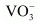Oxidation state of V is + 5.

(ii) Chromate,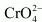Oxidation state of Cr is + 6.

(iii) Permanganate,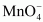Oxidation state of Mn is + 7.

Question7. What is lanthanoid contraction? What are the consequences of lanthanoid contraction?

Solution :
As we move along the lanthanoid series, the atomic number increases gradually by one. This means that the number of electrons and protons present in an atom also increases by one. As electrons are being added to the same shell, the effective nuclear charge increases. This happens because the increase in nuclear attraction due to the addition of proton is more pronounced than the increase in the interelectronic repulsions due to the addition of electron. Also, with the increase in atomic number, the number of electrons in the 4f orbital also increases. The 4f electrons have poor shielding effect. Therefore, the effective nuclear charge experienced by the outer electrons increases. Consequently, the attraction of the nucleus for the outermost electrons increases. This results in a steady decrease in the size of lanthanoids with the increase in the atomic number. This is termed as lanthanoid contraction.

Consequences of lanthanoid contraction

(i) There is similarity in the properties of second and third transition series.

Separation of lanthanoids is possible due to lanthanide contraction.

(iii) It is due to lanthanide contraction that there is variation in the basic strength of lanthanide hydroxides. (Basic strength decreases from La(OH)3 to Lu(OH)3.)

Question8. What are the characteristics of the transition elements and why are they called transition elements? Which of the d-block elements may not be regarded as the transition elements?

Solution :
Transition elements are those elements in which the atoms or ions (in stable oxidation state) contain partially filled d-orbital. These elements lie in the d-block and show a transition of properties between s-block and p-block. Therefore, these are called transition elements.

Elements such as Zn, Cd, and Hg cannot be classified as transition elements because these have completely filled d-subshell.

Question9. In what way is the electronic configuration of the transition elements different from that of the non-transition elements?

Solution :
Transition metals have a partially filled d−orbital. Therefore, the electronic configuration of transition elements is (n − 1)d1-10 ns0-2.

The non-transition elements either do not have a d−orbital or have a fully filled d−orbital. Therefore, the electronic configuration of non-transition elements is ns1-2 or ns2 np1-6.

Question10. What are the different oxidation states exhibited by the lanthanoids?

Solution :
In the lanthanide series, +3 oxidation state is most common i.e., Ln(III) compounds are predominant. However, +2 and +4 oxidation states can also be found in the solution or in solid compounds.

11. Explain giving reasons:

(i) Transition metals and many of their compounds show paramagnetic behaviour.

(ii) The enthalpies of atomisation of the transition metals are high.

(iii) The transition metals generally form coloured compounds.

(iv) Transition metals and their many compounds act as good catalyst.

Solution :
(i) Transition metals show paramagnetic behaviour. Paramagnetism arises due to the presence of unpaired electrons with each electron having a magnetic moment associated with its spin angular momentum and orbital angular momentum. However, in the first transition series, the orbital angular momentum is quenched. Therefore, the resulting paramagnetism is only because of the unpaired electron.

(ii) Transition elements have high effective nuclear charge and a large number of valence electrons. Therefore, they form very strong metallic bonds. As a result, the enthalpy of atomization of transition metals is high.

(iii) Most of the complexes of transition metals are coloured. This is because of the absorption of radiation from visible light region to promote an electron from one of the d−orbitals to another. In the presence of ligands, the d-orbitals split up into two sets of orbitals having different energies. Therefore, the transition of electrons can take place from one set toanother. The energy required for these transitions is quite small and falls in the visible region of radiation. The ions of transition metals absorb the radiation of a particular wavelength and the rest is reflected, imparting colour to the solution.

(iv) The catalytic activity of the transition elements can be explained by two basic facts.

(a) Owing to their ability to show variable oxidation states and form complexes, transition metals form unstable intermediate compounds. Thus, they provide a new path with lower activation energy, Ea, for the reaction.

(b) Transition metals also provide a suitable surface for the reactions to occur.

Question12. What are interstitial compounds? Why are such compounds well known for transition metals?

Solution :
Transition metals are large in size and contain lots of interstitial sites. Transition elements can trap atoms of other elements (that have small atomic size), such as H, C, N, in the interstitial sites of their crystal lattices. The resulting compounds are called interstitial compounds.

Question13. How is the variability in oxidation states of transition metals different from that of the non-transition metals? Illustrate with examples.

Solution :
In transition elements, the oxidation state can vary from +1 to the highest oxidation state by removing all its valence electrons. Also, in transition elements, the oxidation states differ by 1 (Fe2+ and Fe3+; Cu+ and Cu2+). In non-transition elements, the oxidation states differ by 2, for example, +2 and +4 or +3 and +5, etc.

Question14. Describe the preparation of potassium dichromate from iron chromite ore. What is the effect of increasing pH on a solution of potassium dichromate?

Solution :
Potassium dichromate is prepared from chromite ore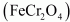in the following steps.

Step (1): Preparation of sodium chromate

Step (2): Conversion of sodium chromate into sodium dichromate

Step(3): Conversion of sodium dichromate to potassium dichromate

Potassium dichromate being less soluble than sodium chloride is obtained in the form of orange coloured crystals and can be removed by filtration.

The dichromate ion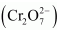exists in equilibrium with chromate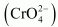ion at pH 4. However, by changing the pH, they can be interconverted.

2CrO24 → 2HCrO4  →2Cr2O72 −

Cr2O72− →2HCrO42−→ 2CrO42−

Question15. Describe the oxidising action of potassium dichromate and write the ionic equations for its reaction with:

(i) iodide (ii) iron(II) solution and (iii) H2S

Solution :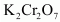acts as a very strong oxidising agent in the acidic medium.takes up electrons to get reduced and acts as an oxidising agent. The reaction of K2Cr2O7 with other iodide, iron (II) solution, and H2S are given below.

(i)oxidizes iodide to iodine.

(ii)oxidizes iron (II) solution to iron (III) solution i.e., ferrous ions to ferric ions.

(iii)oxidizes H2S to sulphur.

Question16. Describe the preparation of potassium permanganate. How does the acidified permanganate solution react with (i) iron(II) ions (ii) SO2 and (iii) oxalic acid?

Write the ionic equations for the reactions.

Solution :
Potassium permanganate can be prepared from pyrolusite (MnO2). The ore is fused with KOH in the presence of either atmospheric oxygen or an oxidising agent, such as KNO3 or KClO4, to give K2MnO4.

The green mass can be extracted with water and then oxidized either electrolytically or by passing chlorine/ozone into the solution.

Electrolytic oxidation

At anode, manganate ions are oxidized to permanganate ions.

Oxidation by chlorine

Oxidation by ozone

(i) Acidified KMnO4 solution oxidizes Fe (II) ions to Fe (III) ions i.e., ferrous ions to ferric ions.

(ii) Acidified potassium permanganate oxidizes SO2 to sulphuric acid.

(iii) Acidified potassium permanganate oxidizes oxalic acid to carbon dioxide.

Question17. For M2+/M and M3+/M2+ systems, thevalues for some metals are as follows:

Cr2+/Cr −0.9V

Cr3/Cr2+ −0.4 V

Mn2+/Mn −1.2V

Mn3+ /Mn2+ +1.5 V

Fe2+ /Fe −0.4V

Fe3+/Fe2+ +0.8 V

Use this data to comment upon:

(i) The stability of Fe3+ in acid solution as compared to that of Cr3+ or Mn3+ and

(ii) The ease with which iron can be oxidised as compared to a similar process for either chromium or manganese metal.

Solution :
(i) Thevalue for Fe3+/Fe2+  is higher than that for Cr3+/Cr2+ and lower than that for Mn3+/Mn2+. So, the reduction of Fe3+ to Fe2+ is easier than the reduction of Mn3+ to Mn2+, but not as easy as the reduction of Cr3+ to Cr2+. Hence, Fe3+ is more stable than Mn3+, but less stable than Cr3+. These metal ions can be arranged in the increasing order of their stability as: Mn3+ < Fe3+ < Cr3+

(ii) The reduction potentials for the given pairs increase in the following order.

Mn2+ / Mn < Cr2+ / Cr < Fe2+ /Fe

So, the oxidation of Fe to Fe2+ is not as easy as the oxidation of Cr to Cr2+ and the oxidation of Mn to Mn2+. Thus, these metals can be arranged in the increasing order of their ability to get oxidised as: Fe < Cr < Mn

Question18. Predict which of the following will be coloured in aqueous solution? Ti3+, V3+, Cu+, Sc3+, Mn2+, Fe3+ and Co2+. Give reasons for each.

Solution :
Only the ions that have electrons in d-orbital and in which d-d transition is possible will be coloured. The ions in which d-orbitals are empty or completely filled will be colourless as no d-d transition is possible in those configurations.

From the above table, it can be easily observed that only Sc3+ has an empty d-orbital and Cu+ has completely filled d-orbitals. All other ions, except Sc3+ and Cu+, will be coloured in aqueous solution because of d−d transitions.

Question19. Compare the stability of +2 oxidation state for the elements of the first transition series.

Solution :

From the above table, it is evident that the maximum number of oxidation states is shown by Mn, varying from +2 to +7. The number of oxidation states increases on moving from Sc to Mn. On moving from Mn to Zn, the number of oxidation states decreases due to a decrease in the number of available unpaired electrons. The relative stability of the +2 oxidation state increases on moving from top to bottom. This is because on moving from top to bottom, it becomes more and more difficult to remove the third electron from the d-orbital.

Question20. Compare the chemistry of actinoids with that of the lanthanoids with special reference to:

(i) electronic configuration (iii) oxidation state

(ii) atomic and ionic sizes and (iv) chemical reactivity.

Solution :
(i) Electronic configuration

The general electronic configuration for lanthanoids is [Xe]54 4f0-14 5d0-1 6s2 and that for actinoids is [Rn]86 5f1-14 6d0-1 7s2. Unlike 4f orbitals, 5f orbitals are not deeply buried and participate in bonding to a greater extent.

(ii) Oxidation states

The principal oxidation state of lanthanoids is (+3). However, sometimes we also encounter oxidation states of + 2 and + 4. This is because of extra stability of fully-filled and half-filled orbitals. Actinoids exhibit a greater range of oxidation states. This is because the 5f, 6d, and 7s levels are of comparable energies. Again, (+3) is the principal oxidation state for actinoids. Actinoids such as lanthanoids have more compounds in +3 state than in +4 state.

(iii) Atomic and lonic sizes

Similar to lanthanoids, actinoids also exhibit actinoid contraction (overall decrease in atomic and ionic radii). The contraction is greater due to the poor shielding effect of 5f orbitals.

(iv) Chemical reactivity

In the lanthanide series, the earlier members of the series are more reactive. They have reactivity that is comparable to Ca. With an increase in the atomic number, the lanthanides start behaving similar to Al. Actinoids, on the other hand, are highly reactive metals, especially when they are finely divided. When they are added to boiling water, they give a mixture of oxide and hydride. Actinoids combine with most of the non-metals at moderate temperatures. Alkalies have no action on these actinoids. In case of acids, they are slightly affected by nitric acid (because of the formation of a protective oxide layer).

Question21. How would you account for the following:

(i) Of the d4species, Cr2+ is strongly reducing while manganese(III) is strongly oxidising.

(ii) Cobalt(II) is stable in aqueous solution but in the presence of complexing reagents it is easily oxidised.

(iii) The d1 configuration is very unstable in ions.

Solution :
(i) Cr2+ is strongly reducing in nature. It has a d4 configuration. While acting as a reducing agent, it gets oxidized to Cr3+ (electronic configuration, d3). This d3 configuration can be written as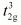configuration, which is a more stable configuration. In the case of Mn3+ (d4), it acts as an oxidizing agent and gets reduced to Mn2+ (d5). This has an exactly half-filled d-orbital and is highly stable.

(ii) Co(II) is stable in aqueous solutions. However, in the presence of strong field complexing reagents, it is oxidized to Co (III). Although the 3rd ionization energy for Co is high, but the higher amount of crystal field stabilization energy (CFSE) released in the presence of strong field ligands overcomes this ionization energy.

(iii) The ions in d1 configuration tend to lose one more electron to get into stable d0 configuration. Also, the hydration or lattice energy is more than sufficient to remove the only electron present in the d-orbital of these ions. Therefore, they act as reducing agents.

Question22. What is meant by ‘disproportionation’? Give two examples of disproportionation reaction in aqueous solution.

Solution :
It is found that sometimes a relatively less stable oxidation state undergoes an oxidation−reduction reaction in which it is simultaneously oxidised and reduced. This is called disproportionation.

For example,

Cr(V) is oxidized to Cr(VI) and reduced to Cr(III).

Mn (VI) is oxidized to Mn (VII) and reduced to Mn (IV).

Question23. Which metal in the first series of transition metals exhibits +1 oxidation state most frequently and why?

Solution :
In the first transition series, Cu exhibits +1 oxidation state very frequently. It is because Cu ( +1) has an electronic configuration of [Ar] 3d10. The completely filled d-orbital makes it highly stable.

Question24. Calculate the number of unpaired electrons in the following gaseous ions: Mn3+, Cr3+, V3+ and Ti3+. Which one of these is the most stable in aqueous solution?

Solution :

Cr3+ is the most stable in aqueous solutions owing to aconfiguration.

Question25. Give examples and suggest reasons for the following features of the transition metal chemistry:

(i)The lowest oxide of transition metal is basic, the highest is amphoteric/acidic.

(ii)A transition metal exhibits highest oxidation state in oxides and fluorides.

(iii) The highest oxidation state is exhibited in oxoanions of a metal.

Solution :
(i) In the case of a lower oxide of a transition metal, the metal atom has a low oxidation state. This means that some of the valence electrons of the metal atom are not involved in bonding. As a result, it can donate electrons and behave as a base.

On the other hand, in the case of a higher oxide of a transition metal, the metal atom has a high oxidation state. This means that the valence electrons are involved in bonding and so, they are unavailable. There is also a high effective nuclear charge.

As a result, it can accept electrons and behave as an acid.

For example,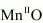is basic and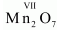is acidic.

(ii) Oxygen and fluorine act as strong oxidising agents because of their high electronegativities and small sizes. Hence, they bring out the highest oxidation states from the transition metals. In other words, a transition metal exhibits higher oxidation states in oxides and fluorides. For example, in OsF6 and V2O5, the oxidation states of Os and V are +6 and +5 respectively.

(iii) Oxygen is a strong oxidising agent due to its high electronegativity and small size. So, oxo-anions of a metal have the highest oxidation state. For example, in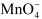, the oxidation state of Mn is +7.

Question26.  Indicate the steps in the preparation of:

(i) K2Cr2O7 from chromite ore.

(ii) KMnO4 from pyrolusite ore.

Solution :
(i) Potassium dichromate (K2Cr2O7) is prepared from chromite ore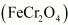in the following steps.

Step (1): Preparation of sodium chromate

Step (2): Conversion of sodium chromate into sodium dichromate

Step(3): Conversion of sodium dichromate to potassium dichromate

Potassium chloride being less soluble than sodium chloride is obtained in the form of orange coloured crystals and can be removed by filtration.

The dichromate ion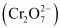exists in equilibrium with chromate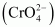ion at pH 4. However, by changing the pH, they can be interconverted.

(ii) Potassium permanganate (KMnO4) can be prepared from pyrolusite (MnO2). The ore is fused with KOH in the presence of either atmospheric oxygen or an oxidising agent, such as KNO3 or KClO4, to give K2MnO4.

The green mass can be extracted with water and then oxidized either electrolytically or by passing chlorine/ozone into the solution.

Electrolytic oxidation

At anode, manganate ions are oxidized to permanganate ions.

Oxidation by chlorine

Oxidation by ozone

Question27. What are alloys? Name an important alloy which contains some of the lanthanoid metals. Mention its uses.

Solution :
An alloy is a solid solution of two or more elements in a metallic matrix. It can either be a partial solid solution or a complete solid solution. Alloys are usually found to possess different physical properties than those of the component elements.

An important alloy of lanthanoids is Mischmetal. It contains lanthanoids (94−95%), iron (5%), and traces of S, C, Si, Ca, and Al.

Uses:

• Mischmetal is used in cigarettes and gas lighters.
• It is used in flame throwing tanks.
• It is used in tracer bullets and shells.

Question28. What are inner transition elements? Decide which of the following atomic numbers are the atomic numbers of the inner transition elements: 29, 59, 74, 95, 102, 104.

Solution :
Inner transition metals are those elements in which the last electron enters the f-orbital. The elements in which the 4f and the 5f orbitals are progressively filled are called f-block elements. Among the given atomic numbers, the atomic numbers of the inner transition elements are 59, 95, and 102.

Question29. The chemistry of the actinoid elements is not so smooth as that of the Lanthanoids. Justify this statement by giving some examples from the oxidation state of these elements.

Solution :
Lanthanoids primarily show three oxidation states (+2, +3, +4). Among these oxidation states, +3 state is the most common. Lanthanoids display a limited number of oxidation states because the energy difference between 4f, 5d, and 6s orbitals is quite large. On the other hand, the energy difference between 5f, 6d, and 7s orbitals is very less. Hence, actinoids display a large number of oxidation states. For example, uranium and plutonium display +3, +4, +5, and +6 oxidation states while neptunium displays +3, +4, +5, and +7. The most common oxidation state in case of actinoids is also +3.

Question30. Which is the last element in the series of the actinoids? Write the electronic configuration of this element. Comment on the possible oxidation state of this element.

Solution :
The last element in the actinoid series is lawrencium, Lr. Its atomic number is 103 and its electronic configuration is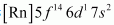. The most common oxidation state displayed by it is +3; because after losing 3 electrons it attains stable f14 configuration.

Question31. Use Hund’s rule to derive the electronic configuration of Ce3+ ion and calculate its magnetic moment on the basis of ‘spin-only’ formula.

Solution :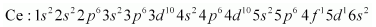Magnetic moment can be calculated as:

μ = √n(n + 2)

Where,

n = number of unpaired electrons The electronic configuration of Ce3+ : 1s22s22p63s23p63d104s24p64d105s25p64f1

In Ce3+, n = 1

∴ μ = √1 ( 1 +2 )= √3 = 1.732 B.M

Question32. Name the members of the lanthanoid series which exhibit +4 oxidation state and those which exhibit +2 oxidation state. Try to correlate this type of behavior with the electronic configurations of these elements.

Solution :
The lanthanides that exhibit +2 and +4 states are shown in the given table. The atomic numbers of the elements are given in the parenthesis.

Ce after forming Ce4+ attains a stable electronic configuration of [Xe].

Tb after forming Tb4+ attains a stable electronic configuration of [Xe] 4f7.

Eu after forming Eu2+ attains a stable electronic configuration of [Xe] 4f7.

Yb after forming Yb2+ attains a stable electronic configuration of [Xe] 4f14.

Question33. Compare the chemistry of the actinoids with that of lanthanoids with reference to:

(i) electronic configuration

(ii) oxidation states and

(iii) chemical reactivity.

Solution :
Electronic configuration:

The general electronic configuration for lanthanoids is [Xe]54 4f0-14 5d0-1 6s2 and that for actinoids is [Rn]86 5f1-14 6d0-1 7s2. Unlike 4f orbitals, 5f orbitals are not deeply buried and participate in bonding to a greater extent.

Oxidation states:

The principal oxidation state of lanthanoids is (+3). However, sometimes we also encounter oxidation states of + 2 and + 4. This is because of extra stability of fully-filled and half-filled orbitals. Actinoids exhibit a greater range of oxidation states. This is because the 5f, 6d, and 7s levels are of comparable energies. Again, (+3) is the principal oxidation state for actinoids. Actinoids such as lanthanoids have more compounds in +3 state than in +4 state.

Chemical reactivity:

In the lanthanide series, the earlier members of the series are more reactive. They have reactivity that is comparable to Ca. With an increase in the atomic number, the lanthanides start behaving similar to Al. Actinoids, on the other hand, are highly reactive metals, especially when they are finely divided. When they are added to boiling water, they give a mixture of oxide and hydride. Actinoids combine with most of the non-metals at moderate temperatures. Alkalies have no action on these actinoids. In case of acids, they are slightly affected by nitric acid (because of the formation of a protective oxide layer).

Question34. Write the electronic configurations of the elements with the atomic numbers 61, 91, 101, and 109.

Solution :

Question35. Compare the general characteristics of the first series of the transition metals with those of the second and third series metals in the respective vertical columns. Give special emphasis on the following points:

(i) electronic configurations,

(ii) oxidation states,

(iii) ionisation enthalpies, and

(iv) atomic sizes.

Solution :
(i) In the 1st, 2nd and 3rd transition series, the 3d, 4d and 5d orbitals are respectively filled.

We know that elements in the same vertical column generally have similar electronic configurations.

In the first transition series, two elements show unusual electronic configurations:

Similarly, there are exceptions in the second transition series. These are:

There are some exceptions in the third transition series as well. These are:

As a result of these exceptions, it happens many times that the electronic configurations of the elements present in the same group are dissimilar.

(ii) In each of the three transition series the number of oxidation states shown by the elements is the maximum in the middle and the minimum at the extreme ends.

However, +2 and +3 oxidation states are quite stable for all elements present in the first transition series. All metals present in the first transition series form stable compounds in the +2 and +3 oxidation states. The stability of the +2 and +3 oxidation states decreases in the second and the third transition series, wherein higher oxidation states are more important.

For example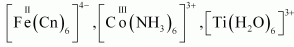are stable complexes, but no such complexes are known for the second and third transition series such as Mo, W, Rh, In. They form complexes in which their oxidation states are high. For example: WCl6, ReF7, RuO4, etc.

(iii) In each of the three transition series, the first ionisation enthalpy increases from left to right. However, there are some exceptions. The first ionisation enthalpies of the third transition series are higher than those of the first and second transition series. This occurs due to the poor shielding effect of 4f electrons in the third transition series.

Certain elements in the second transition series have higher first ionisation enthalpies than elements corresponding to the same vertical column in the first transition series. There are also elements in the 2nd transition series whose first ionisation enthalpies are lower than those of the elements corresponding to the same vertical column in the 1st transition series.

(iv) Atomic size generally decreases from left to right across a period. Now, among the three transition series, atomic sizes of the elements in the second transition series are greater than those of the elements corresponding to the same vertical column in the first transition series. However, the atomic sizes of the elements in the third transition series are virtually the same as those of the corresponding members in the second transition series. This is due to lanthanoid contraction.

Question36. Write down the number of 3d electrons in each of the following ions:

Ti2+, V2+, Cr3+, Mn2+, Fe2+, Fe3+, CO2+, Ni2+ and Cu2+.

Indicate how would you expect the five 3d orbitals to be occupied for these hydrated ions (octahedral).

Solution :

Question37. Comment on the statement that elements of the first transition series possess many properties different from those of heavier transition elements.

Solution :
The properties of the elements of the first transition series differ from those of the heavier transition elements in many ways.

(i) The atomic sizes of the elements of the first transition series are smaller than those of the heavier elements (elements of 2nd and 3rd transition series).

However, the atomic sizes of the elements in the third transition series are virtually the same as those of the corresponding members in the second transition series. This is due to lanthanoid contraction.

(ii) +2 and +3 oxidation states are more common for elements in the first transition series, while higher oxidation states are more common for the heavier elements.

(iii) The enthalpies of atomisation of the elements in the first transition series are lower than those of the corresponding elements in the second and third transition series.

(iv) The melting and boiling points of the first transition series are lower than those of the heavier transition elements. This is because of the occurrence of stronger metallic bonding (M−M bonding).

(v) The elements of the first transition series form low-spin or high-spin complexes depending upon the strength of the ligand field. However, the heavier transition elements form only low-spin complexes, irrespective of the strength of the ligand field.

Question38. What can be inferred from the magnetic moment values of the following complex species?

Example Magnetic Moment (BM)

K4[Mn(CN)6] 2.2

[Fe(H2O)6]2+ 5.3

K2[MnCl4] 5.9

Solution :
Magnetic moment (μ ) is given as μ = √n(n + 2).

For value n = 1, μ = √1(1 + 2) = √3 = 1.73

For value n = 2, μ = √2(2 + 2) = √8 = 2.83

For value n = 3, μ = √3(3 + 2) = √15 = 3.87

For value n = 4, μ = √4(4 + 2) = √24 = 4.899

For value n = 5, μ = √5(5 + 2) = √35 = 5.92

(i) K4[Mn(CN)6]

For in transition metals, the magnetic moment is calculated from the spin-only formula. Therefore,

√n(n + 2) = 2.2

We can see from the above calculation that the given value is closest to n= 1. Also, in this complex, Mn is in the +2 oxidation state. This means that Mn has 5 electrons in the d-orbital.

Hence, we can say that CN− is a strong field ligand that causes the pairing of electrons.

(ii) [Fe(H2O)6]2+

√n(n + 2) = 5.3

We can see from the above calculation that the given value is closest to n = 4. Also, in this complex, Fe is in the +2 oxidation state. This means that Fe has 6 electrons in the d-orbital.

Hence, we can say that H2O is a weak field ligand and does not cause the pairing of electrons.

(iii) K2[MnCl4]

√n(n + 2) = 5.9

We can see from the above calculation that the given value is closest to n= 5. Also, in this complex, Mn is in the +2 oxidation state. This means that Mn has 5 electrons in the d-orbital.

Hence, we can say that Cl− is a weak field ligand and does not cause the pairing of electrons.

Question39. Silver atom has completely filled d orbitals (4d10) in its ground state. How can you say that it is a transition element?

Solution :
Ag has a completely filled 4d orbital (4d10 5s1) in its ground state. Now, silver displays two oxidation states (+1 and +2). In the +1 oxidation state, an electron is removed from the s-orboital. However, in the +2 oxidation state, an electron is removed from the d-orbital. Thus, the d-orbital now becomes incomplete (4d9). Hence, it is a transition element.

Question40. In the series Sc (Z = 21) to Zn (Z = 30), the enthalpy of atomization of zinc is the lowest, i.e., 126 kJ mol−1. Why?

Solution :
The extent of metallic bonding an element undergoes decides the enthalpy of atomization. The more extensive the metallic bonding of an element, the more will be its enthalpy of atomization. In all transition metals (except Zn, electronic configuration: 3d10 4s2), there are some unpaired electrons that account for their stronger metallic bonding. Due to the absence of these unpaired electrons, the inter-atomic electronic bonding is the weakest in Zn and as a result, it has the least enthalpy of atomization.

Question41.  Which of the 3d series of the transition metals exhibits the largest number of oxidation states and why?

Solution :
Mn (Z = 25) = 3d5 4s2

Mn has the maximum number of unpaired electrons present in the d-subshell (5 electrons). Hence, Mn exhibits the largest number of oxidation states, ranging from +2 to +7.

Question42. The Eθ(M2+/M) value for copper is positive (+0.34V). What is possibly the reason for this? (Hint: consider its high ΔaHθ and low ΔhydHθ)

Solution :
The Eθ(M2+/M) value of a metal depends on the energy changes involved in the following:

1. Sublimation: The energy required for converting one mole of an atom from the solid state to the gaseous state.

2. Ionization: The energy required to take out electrons from one mole of atoms in the gaseous state.

3. Hydration: The energy released when one mole of ions are hydrated.

Now, copper has a high energy of atomization and low hydration energy. Hence, the Eθ(M2+/M) value for copper is positive.

Question43.. How would you account for the irregular variation of ionization enthalpies (first and second) in the first series of the transition elements?

Solution :
Ionization enthalpies are found to increase in the given series due to a continuous filling of the inner d-orbitals. The irregular variations of ionization enthalpies can be attributed to the extra stability of configurations such as d0, d5, d10. Since these states are exceptionally stable, their ionization enthalpies are very high.

In case of first ionization energy, Cr has low ionization energy. This is because after losing one electron, it attains the stable configuration (3d5). On the other hand, Zn has exceptionally high first ionization energy as an electron has to be removed from stable and fully-filled orbitals (3d10 4s2).

Second ionization energies are higher than the first since it becomes difficult to remove an electron when an electron has already been taken out. Also, elements like Cr and Cu have exceptionally high second ionization energies as after losing the first electron, they have attained the stable configuration (Cr+: 3d5 and Cu+: 3d10). Hence, taking out one electron more from this stable configuration will require a lot of energy.

Question44. Why is the highest oxidation state of a metal exhibited in its oxide or fluoride only?

Solution :
Both oxide and fluoride ions are highly electronegative and have a very small size. Due to these properties, they are able to oxidize the metal to its highest oxidation state.

Question45. Which is a stronger reducing agent Cr2+ or Fe2+ and why?

Solution :
The following reactions are involved when Cr2+ and Fe2+ act as reducing agents.

Cr2+ → Cr3+ Fe2+ →  Fe3+

The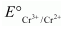value is −0.41 V and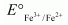is +0.77 V. This means that Cr2+ can be easily oxidized to Cr3+, but Fe2+ does not get oxidized to Fe3+ easily. Therefore, Cr2+ is a better reducing agent that Fe3+.

Question46. Calculate the ‘spin only’ magnetic moment of M2+(aq) ion (Z = 27).

Solution :

Question47. Explain why Cu+ ion is not stable in aqueous solutions?

Solution :
In an aqueous medium, Cu2+ is more stable than Cu+. This is because although energy is required to remove one electron from Cu+ to Cu2+, high hydration energy of Cu2+ compensates for it. Therefore, Cu+ ion in an aqueous solution is unstable. It disproportionates to give Cu2+ and Cu.

Question48.  Actinoid contraction is greater from element to element than lanthanoid contraction. Why?

Solution :
In actinoids, 5f orbitals are filled. These 5f orbitals have a poorer shielding effect than 4f orbitals (in lanthanoids). Thus, the effective nuclear charge experienced by electrons in valence shells in case of actinoids is much more that that experienced by lanthanoids. Hence, the size contraction in actinoids is greater as compared to that in lanthanoids.

Get 30% off your first purchase!

X
error: Content is protected !!
Scroll to Top